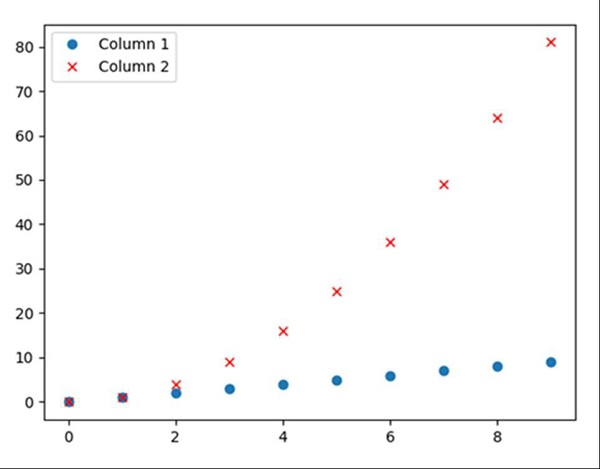Save the plots into a PDF in matplotlib

MatplotlibServer Side ProgrammingProgramming

Using plt.savefig("myImagePDF.pdf", format="pdf", bbox_inches="tight") method, we can save a figure in PDF format.

Steps

• Create a dictionary with Column 1 and Column 2 as the keys and Values are like i and i*i, where i is from 0 to 10, respectively.

• Create a data frame using pd.DataFrame(d), d created in step 1.

• Plot the data frame with ‘o’ and ‘rx’ style.

• To save the file in PDF format, use savefig() method where the image name is myImagePDF.pdf, format = ”pdf”.

• To show the image, use the plt.show() method.

Example

import pandas as pd
from matplotlib import pyplot as plt

d = {'Column 1': [i for i in range(10)], 'Column 2': [i * i for i in range(10)]}

df = pd.DataFrame(d)

df.plot(style=['o', 'rx'])
plt.savefig("myImagePDF.pdf", format="pdf", bbox_inches="tight")
plt.show()

Output